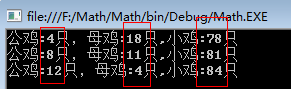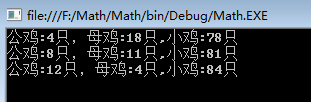#0
0
01. 云栖社区>
2. 博客>
3. 正文

## 经典算法题每日演练——第一题 百钱买百鸡

百钱买百鸡的问题算是一套非常经典的不定方程的问题，题目很简单：公鸡5文钱一只，母鸡3文钱一只，小鸡3只一文钱，

可以得出如下的不定方程，

x+y+z=100,

5x+3y+z/3=100，

下面再看看x，y，z的取值范围。

由于只有100文钱，则5x<100 => 0<x<20, 同理  0<y<33,那么z=100-x-y，

好，我们已经分析清楚了，下面就可以编码了。

``` 1     class Program
2     {
3         static void Main(string[] args)
4         {
5             //公鸡的上线
6             for (int x = 1; x < 20; x++)
7             {
8                 //母鸡的上线
9                 for (int y = 1; y < 33; y++)
10                 {
11                     //剩余小鸡
12                     var z = 100 - x - y;
13
14                     if ((z % 3 == 0) && (x * 5 + y * 3 + z / 3 == 100))
15                     {
16                         Console.WriteLine("公鸡:{0}只，母鸡:{1}只,小鸡:{2}只", x, y, z);
17                     }
18                 }
19             }
21         }
22     }```x+y+z=100          ①

5x+3y+z/3=100    ②

令②x3-① 可得

7x+4y=100

=>y=25-(7/4)x          ③

x=4k                    ④

=> y=25-7k               ⑤

=> z=75+3k               ⑥

``` 1     class Program
2     {
3         static void Main(string[] args)
4         {
5             int x, y, z;
6
7             for (int k = 1; k <= 3; k++)
8             {
9                 x = 4 * k;
10                 y = 25 - 7 * k;
11                 z = 75 + 3 * k;
12
13                 Console.WriteLine("公鸡:{0}只，母鸡:{1}只,小鸡:{2}只", x, y, z);
14             }
15
17         }
18     }```+ 关注